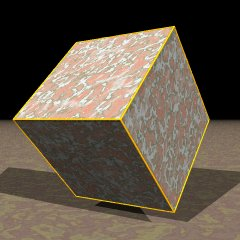Acronym x4oPo Name family of (generally hyperbolic) order P square tilings©© Vertex figure [4P] = qPo Especially cube (P=3, spherical)   squat (P=4, euclidean)   x4o5o (P=5)   x4o6o (P=6)   x4o8o (P=8)   x4o∞o (P→∞)   x4o2Qo (P even) ExternallinksIncidence matrix according to Dynkin symbol

x4oPo   (P parametrisable, N → ∞)

. . . | 4N |   P |  P
------+----+-----+---
x . . |  2 | 2PN |  2
------+----+-----+---
x4o . |  4 |   4 | PN

snubbed forms: s4oPo